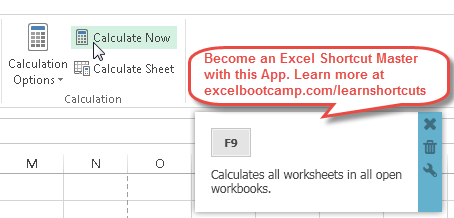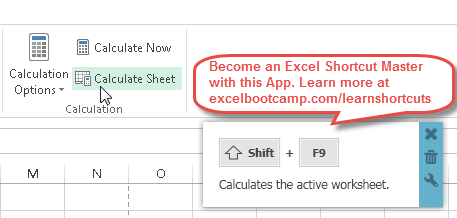# Excel Refresh or Calculate Shortcut

### Calculate Part of a Formula

While in Cell Edit Mode, this Excel Shortcut calculates part of a formula.

PC Shorcut:F9
Mac Shorcut:fn+F9
Remember This Shortcut:
F9 is also the standard shortcut to calculate. So, within a cell F9 calculates just the selected piece.

### Calculate Now

This Excel Shortcut calculates the active Excel workbooks.PC Shorcut:F9
Mac Shorcut:fn+F9
Remember This Shortcut:
F9 is the universal shortcut for refresh

### Calculate Active Sheet

This Excel Shortcut calculates the active worksheet.PC Shorcut:Shift+F9
Mac Shorcut:fn++F9
Remember This Shortcut:
F9 is the universal shortcut for refresh

### Force Calculate

This Excel Shortcut forces the active Excel workbooks to recalculate even if cells have not been changed.

PC Shorcut:Ctrl+ALT+F9
Mac Shorcut:n/a
Remember This Shortcut:
F9 is the universal shortcut for refresh

### Refresh

This Excel Shortcut calculates the active Excel workbooks.PC Shorcut:F9
Mac Shorcut:fn+F9
Remember This Shortcut:
F9 is the universal shortcut for refresh